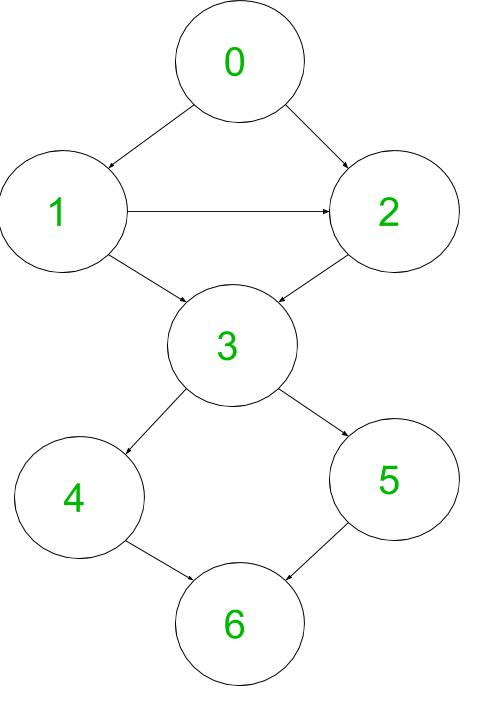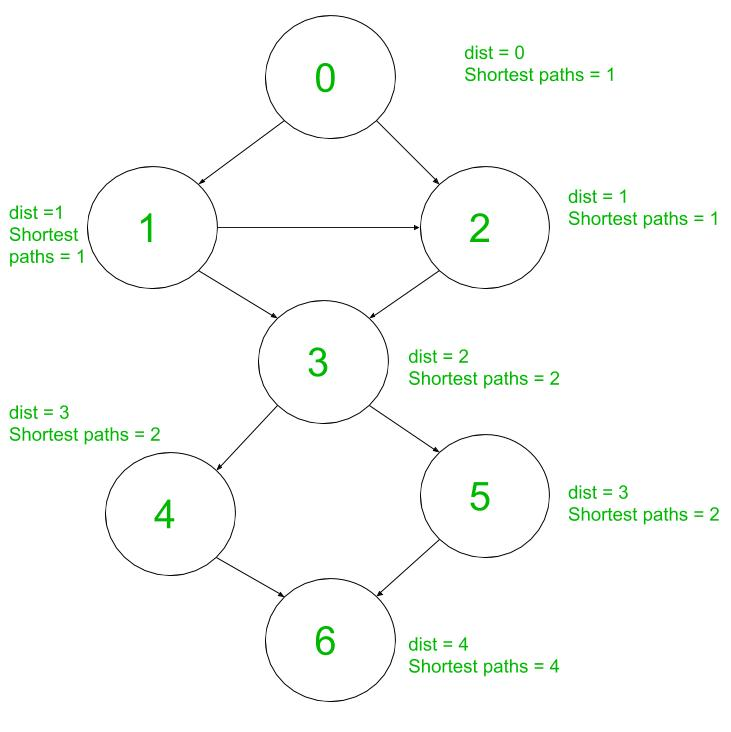# Number of shortest paths in an unweighted and directed graph

• Difficulty Level : Hard
• Last Updated : 21 Aug, 2021

Given an unweighted directed graph, can be cyclic or acyclic. Print the number of shortest paths from a given vertex to each of the vertices. For example consider the below graph. There is one shortest path vertex 0 to vertex 0 (from each vertex there is a single shortest path to itself), one shortest path between vertex 0 to vertex 2 (0->2), and there are 4 different shortest paths from vertex 0 to vertex 6:
1. 0->1->3->4->6
2. 0->1->3->5->6
3. 0->2->3->4->6
4. 0->2->3->5->6The idea is to use BFS. We use two arrays called dist[] and paths[], dist[] represents the shortest distances from source vertex, and paths[] represents the number of different shortest paths from the source vertex to each of the vertices. Initially all the elements in dist[] are infinity except source vertex which is equal to 0, since the distance to source vertex from itself is 0, and all the elements in paths[] are 0 except source vertex which is equal to 1, since each vertex has a single shortest path to itself. after that, we start traversing the graph using BFS manner.
Then, for every neighbor Y of each vertex X do:
1) if dist[Y] > dist[X]+1 decrease the dist[Y] to dist[X] +1 and assign the number of paths of vertex X to number of paths of vertex Y.
2) else if dist[Y] = dist[X] + 1, then add the number of paths of vertex X to the number of paths of vertex Y.
For example:
Let’s take a look at the below graph. The source vertex is 0. Suppose we traverse on vertex 2, we check all its neighbors, which is only 3.since vertex 3 was already visited when we were traversed vertex 1, dist = 2 and paths = 1. The second condition is true, so it means that additional shortest paths have been found, so we add to the number of paths of vertex 3, the number of paths of vertex 2.
The equal condition happens when we traverse on vertex 5:## C++

 `// CPP program to count number of shortest` `// paths from a given source to every other` `// vertex using BFS.` `#include ` `using` `namespace` `std;`   `// Traverses graph in BFS manner. It fills ` `// dist[] and paths[]` `void` `BFS(vector<``int``> adj[], ``int` `src, ``int` `dist[],` `                           ``int` `paths[], ``int` `n)` `{` `    ``bool` `visited[n];` `    ``for` `(``int` `i = 0; i < n; i++)` `        ``visited[i] = ``false``;` `    ``dist[src] = 0;` `    ``paths[src] = 1;`   `    ``queue <``int``> q;` `    ``q.push(src);` `    ``visited[src] = ``true``;` `    ``while` `(!q.empty())` `    ``{` `        ``int` `curr = q.front();` `        ``q.pop();`   `        ``// For all neighbors of current vertex do:` `        ``for` `(``auto` `x : adj[curr])` `        ``{` `            ``// if the current vertex is not yet` `            ``// visited, then push it to the queue.` `            ``if` `(visited[x] == ``false``)` `            ``{` `                ``q.push(x);` `                ``visited[x] = ``true``;` `            ``}`   `            ``// check if there is a better path.` `            ``if` `(dist[x] > dist[curr] + 1)` `            ``{` `                ``dist[x] = dist[curr] + 1;` `                ``paths[x] = paths[curr];` `            ``}`   `            ``// additional shortest paths found` `            ``else` `if` `(dist[x] == dist[curr] + 1)` `                ``paths[x] += paths[curr];` `        ``}` `    ``}` `}`   `// function to find number of different` `// shortest paths form given vertex s.` `// n is number of vertices.` `void` `findShortestPaths(vector<``int``> adj[],` `                       ``int` `s, ``int` `n)` `{` `    ``int` `dist[n], paths[n];` `    ``for` `(``int` `i = 0; i < n; i++)` `        ``dist[i] = INT_MAX;` `    ``for` `(``int` `i = 0; i < n; i++)` `        ``paths[i] = 0;` `    ``BFS(adj, s, dist, paths, n);` `    ``cout << ``"Numbers of shortest Paths are: "``;` `    ``for` `(``int` `i = 0; i < n; i++)` `        ``cout << paths[i] << ``" "``;` `}`   `// A utility function to add an edge in a` `// directed graph.` `void` `addEdge(vector<``int``> adj[], ``int` `u, ``int` `v)` `{` `    ``adj[u].push_back(v);` `}`   `// Driver code` `int` `main()` `{` `    ``int` `n = 7; ``// Number of vertices` `    ``vector <``int``> adj[n];` `    ``addEdge(adj, 0, 1);` `    ``addEdge(adj, 0, 2);` `    ``addEdge(adj, 1, 2);` `    ``addEdge(adj, 1, 3);` `    ``addEdge(adj, 2, 3);` `    ``addEdge(adj, 3, 4);` `    ``addEdge(adj, 3, 5);` `    ``addEdge(adj, 4, 6);` `    ``addEdge(adj, 5, 6);` `    ``findShortestPaths(adj, 0, 7);` `    ``return` `0;` `}`

## Java

 `// Java program to count number of shortest ` `// paths from a given source to every other ` `// vertex using BFS.` `import` `java.io.*;` `import` `java.util.*;`   `class` `GFG ` `{`   `    ``// Traverses graph in BFS manner. ` `    ``// It fills dist[] and paths[]` `    ``static` `void` `BFS(Vector[] adj, ``int` `src,` `                  ``int` `dist[], ``int` `paths[], ``int` `n) ` `    ``{` `        ``boolean``[] visited = ``new` `boolean``[n];` `        ``for` `(``int` `i = ``0``; i < n; i++)` `            ``visited[i] = ``false``;` `        ``dist[src] = ``0``;` `        ``paths[src] = ``1``;`   `        ``Queue q = ``new` `LinkedList<>();` `        ``q.add(src);` `        ``visited[src] = ``true``;` `        ``while` `(!q.isEmpty())` `        ``{` `            ``int` `curr = q.peek();` `            ``q.poll();`   `            ``// For all neighbors of current vertex do:` `            ``for` `(``int` `x : adj[curr])` `            ``{`   `                ``// if the current vertex is not yet` `                ``// visited, then push it to the queue.` `                ``if` `(visited[x] == ``false``)` `                ``{` `                    ``q.add(x);` `                    ``visited[x] = ``true``;` `                ``}`   `                ``// check if there is a better path.` `                ``if` `(dist[x] > dist[curr] + ``1``) ` `                ``{` `                    ``dist[x] = dist[curr] + ``1``;` `                    ``paths[x] = paths[curr];` `                ``}`   `                ``// additional shortest paths found` `                ``else` `if` `(dist[x] == dist[curr] + ``1``)` `                    ``paths[x] += paths[curr];` `            ``}` `        ``}` `    ``}`   `    ``// function to find number of different` `    ``// shortest paths form given vertex s.` `    ``// n is number of vertices.` `    ``static` `void` `findShortestPaths(Vector adj[], ` `                                           ``int` `s, ``int` `n) ` `    ``{` `        ``int``[] dist = ``new` `int``[n], paths = ``new` `int``[n];` `        `  `        ``for` `(``int` `i = ``0``; i < n; i++)` `            ``dist[i] = Integer.MAX_VALUE;`   `        ``for` `(``int` `i = ``0``; i < n; i++)` `            ``paths[i] = ``0``;`   `        ``BFS(adj, s, dist, paths, n);`   `        ``System.out.print(``"Numbers of shortest Paths are: "``);` `        ``for` `(``int` `i = ``0``; i < n; i++)` `            ``System.out.print(paths[i] + ``" "``);` `    ``}`   `    ``// A utility function to add an edge in a` `    ``// directed graph.` `    ``static` `void` `addEdge(Vector adj[],` `                                 ``int` `u, ``int` `v)` `    ``{` `        ``adj[u].add(v);` `    ``}`   `    ``// Driver Code` `    ``public` `static` `void` `main(String[] args) ` `    ``{` `        ``int` `n = ``7``; ``// Number of vertices`   `        ``Vector[] adj = ``new` `Vector[n];` `        ``for` `(``int` `i = ``0``; i < n; i++)` `            ``adj[i] = ``new` `Vector<>();`   `        ``addEdge(adj, ``0``, ``1``);` `        ``addEdge(adj, ``0``, ``2``);` `        ``addEdge(adj, ``1``, ``2``);` `        ``addEdge(adj, ``1``, ``3``);` `        ``addEdge(adj, ``2``, ``3``);` `        ``addEdge(adj, ``3``, ``4``);` `        ``addEdge(adj, ``3``, ``5``);` `        ``addEdge(adj, ``4``, ``6``);` `        ``addEdge(adj, ``5``, ``6``);` `        ``findShortestPaths(adj, ``0``, ``7``);` `    ``}` `}`   `// This code is contributed by` `// sanjeev2552`

## Python3

 `# Python3 program to count number of shortest` `# paths from a given source to every other` `# vertex using BFS.` `from` `collections ``import` `deque` `from` `sys ``import` `maxsize as INT_MAX`   `# Traverses graph in BFS manner. It fills` `# dist[] and paths[]` `def` `BFS(adj: ``list``, src: ``int``, dist: ``list``, paths: ``list``, n: ``int``):` `    ``visited ``=` `[``False``] ``*` `n` `    ``dist[src] ``=` `0` `    ``paths[src] ``=` `1`   `    ``q ``=` `deque()` `    ``q.append(src)` `    ``visited[src] ``=` `True` `    ``while` `q:` `        ``curr ``=` `q[``0``]` `        ``q.popleft()`   `        ``# For all neighbors of current vertex do:` `        ``for` `x ``in` `adj[curr]:`   `            ``# if the current vertex is not yet` `            ``# visited, then push it to the queue.` `            ``if` `not` `visited[x]:` `                ``q.append(x)` `                ``visited[x] ``=` `True`   `            ``# check if there is a better path.` `            ``if` `dist[x] > dist[curr] ``+` `1``:` `                ``dist[x] ``=` `dist[curr] ``+` `1` `                ``paths[x] ``=` `paths[curr]`   `            ``# additional shortest paths found` `            ``elif` `dist[x] ``=``=` `dist[curr] ``+` `1``:` `                ``paths[x] ``+``=` `paths[curr]`   `# function to find number of different` `# shortest paths form given vertex s.` `# n is number of vertices.` `def` `findShortestPaths(adj: ``list``, s: ``int``, n: ``int``):` `    ``dist ``=` `[INT_MAX] ``*` `n` `    ``paths ``=` `[``0``] ``*` `n` `    ``BFS(adj, s, dist, paths, n)` `    ``print``(``"Numbers of shortest Paths are:"``, end``=``" "``)` `    ``for` `i ``in` `paths:` `        ``print``(i, end``=``" "``)`   `# A utility function to add an edge in a` `# directed graph.` `def` `addEdge(adj: ``list``, u: ``int``, v: ``int``):` `    ``adj[u].append(v)`   `# Driver Code` `if` `__name__ ``=``=` `"__main__"``:`   `    ``n ``=` `7` `# Number of vertices` `    ``adj ``=` `[``0``] ``*` `n` `    ``for` `i ``in` `range``(n):` `        ``adj[i] ``=` `[]` `    ``addEdge(adj, ``0``, ``1``)` `    ``addEdge(adj, ``0``, ``2``)` `    ``addEdge(adj, ``1``, ``2``)` `    ``addEdge(adj, ``1``, ``3``)` `    ``addEdge(adj, ``2``, ``3``)` `    ``addEdge(adj, ``3``, ``4``)` `    ``addEdge(adj, ``3``, ``5``)` `    ``addEdge(adj, ``4``, ``6``)` `    ``addEdge(adj, ``5``, ``6``)` `    ``findShortestPaths(adj, ``0``, ``7``)`   `# This code is contributed by` `# sanjeev2552`

## C#

 `// C# program to count number of shortest ` `// paths from a given source to every other ` `// vertex using BFS.` `using` `System;` `using` `System.Collections.Generic;`   `class` `GFG ` `{`   `    ``// Traverses graph in BFS manner. ` `    ``// It fills dist[] and paths[]` `    ``static` `void` `BFS(List<``int``>[] adj, ``int` `src,` `                ``int` `[]dist, ``int` `[]paths, ``int` `n) ` `    ``{` `        ``bool``[] visited = ``new` `bool``[n];` `        ``for` `(``int` `i = 0; i < n; i++)` `            ``visited[i] = ``false``;` `        ``dist[src] = 0;` `        ``paths[src] = 1;`   `        ``List<``int``> q = ``new` `List<``int``>();` `        ``q.Add(src);` `        ``visited[src] = ``true``;` `        ``while` `(q.Count != 0)` `        ``{` `            ``int` `curr = q;` `            ``q.RemoveAt(0);`   `            ``// For all neighbors of current vertex do:` `            ``foreach` `(``int` `x ``in` `adj[curr])` `            ``{`   `                ``// if the current vertex is not yet` `                ``// visited, then push it to the queue.` `                ``if` `(visited[x] == ``false``)` `                ``{` `                    ``q.Add(x);` `                    ``visited[x] = ``true``;` `                ``}`   `                ``// check if there is a better path.` `                ``if` `(dist[x] > dist[curr] + 1) ` `                ``{` `                    ``dist[x] = dist[curr] + 1;` `                    ``paths[x] = paths[curr];` `                ``}`   `                ``// additional shortest paths found` `                ``else` `if` `(dist[x] == dist[curr] + 1)` `                    ``paths[x] += paths[curr];` `            ``}` `        ``}` `    ``}`   `    ``// function to find number of different` `    ``// shortest paths form given vertex s.` `    ``// n is number of vertices.` `    ``static` `void` `findShortestPaths(List<``int``> []adj, ` `                                        ``int` `s, ``int` `n) ` `    ``{` `        ``int``[] dist = ``new` `int``[n], paths = ``new` `int``[n];` `        `  `        ``for` `(``int` `i = 0; i < n; i++)` `            ``dist[i] = ``int``.MaxValue;`   `        ``for` `(``int` `i = 0; i < n; i++)` `            ``paths[i] = 0;`   `        ``BFS(adj, s, dist, paths, n);`   `        ``Console.Write(``"Numbers of shortest Paths are: "``);` `        ``for` `(``int` `i = 0; i < n; i++)` `            ``Console.Write(paths[i] + ``" "``);` `    ``}`   `    ``// A utility function to add an edge in a` `    ``// directed graph.` `    ``static` `void` `addEdge(List<``int``> []adj,` `                                ``int` `u, ``int` `v)` `    ``{` `        ``adj[u].Add(v);` `    ``}`   `    ``// Driver Code` `    ``public` `static` `void` `Main(String[] args) ` `    ``{` `        ``int` `n = 7; ``// Number of vertices`   `        ``List<``int``>[] adj = ``new` `List<``int``>[n];` `        ``for` `(``int` `i = 0; i < n; i++)` `            ``adj[i] = ``new` `List<``int``>();`   `        ``addEdge(adj, 0, 1);` `        ``addEdge(adj, 0, 2);` `        ``addEdge(adj, 1, 2);` `        ``addEdge(adj, 1, 3);` `        ``addEdge(adj, 2, 3);` `        ``addEdge(adj, 3, 4);` `        ``addEdge(adj, 3, 5);` `        ``addEdge(adj, 4, 6);` `        ``addEdge(adj, 5, 6);` `        ``findShortestPaths(adj, 0, 7);` `    ``}` `}`   `// This code is contributed by 29AjayKumar`

## Javascript

 ``

Output:

`Numbers of shortest Paths are: 1 1 1 2 2 2 4`

Time Complexity : O(V + E)

My Personal Notes arrow_drop_up
Recommended Articles
Page :